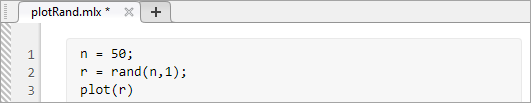## 保存和备份代码

### 保存代码### 备份代码

• 正在编辑的文件备份副本的频率。

• 创建文件备份副本时使用的文件扩展名。

• 保存文件备份副本的位置。

• 在编辑器中关闭对应的源文件时，是否自动删除文件的备份副本。

MATLAB Online 中，每次在编辑器中保存代码文件时，MATLAB 都会将代码文件的内容存储在版本历史记录中。有关还原在 MATLAB Online 中文件的以前版本的详细信息，请参阅Access Files in MATLAB Online

MATLAB 不会自动创建在实时编辑器中修改的文件的备份。

### 有关保存文件的建议

MathWorks® 建议您将创建的文件保存到 `matlabroot` 文件夹树之外的文件夹中，其中 `matlabroot` 是您在命令行窗口中键入 `matlabroot` 时返回的文件夹。同样，当您编辑从 MathWorks 获得的文件时，请将编辑后的版本保存在 `matlabroot` 文件夹树之外。如果您将文件保存在 `matlabroot` 文件夹树中，则当您安装新版本的 MATLAB 时，这些文件可能会被覆盖。